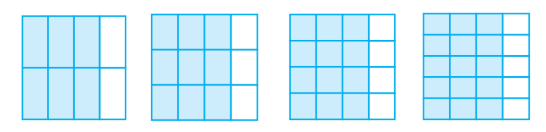## Filters

Sort by :
Q

3.20 b) Given the resistances of 1 Ω, 2 Ω, 3 Ω, how will be combine them to get an equivalent resistance of (iii) 6 Ω

1 Ω+2 Ω+ 3 Ω= 6 Ω, so we will combine the resistance in series.

Question 6. Let ∗ be the binary operation on N given by a ∗ b = L.C.M. of a and b. Find

(v) Which elements of N are invertible for the operation ∗?

An element a is invertible in N  if  Here a is inverse of b. a*b=1=b*a a*b=L.C.M. od a and b a=b=1 So 1 is the only invertible element of N

Write these in ascending and also in descending order.

(a)

(b)

(c)

option A

Which is the larger fraction?

(i)   or

(ii)   or

(iii)   or

The fractions are shown below using greater than or less than sign                      (i)                           (ii)                         (iii)

You get one-fifth of a bottle of juice and your sister gets one-third of the same size of a bottle of juice. Who gets more?

one third is greater potion than one fifth

Is in its simplest form?

Yes,  is in its simplest form because 49 and 64 has no common divisor.

Write the simplest form of :

(i)

(ii)

(iii)

(iv)

(v)

A

Find five equivalent fractions of each of the following:

(i)

(ii)

(iii)

(iv)

(i)                 (ii)                 (iii)                 (iv)

Identify the fractions in each. Are these fractions equivalent?(i)                                       (ii)                          (iii)                              (iv)  all fractions in it simplest form is   So all fractions are equivalent

Give example of four equivalent fractions.

Four example of  equivalent fractions are :

Are and ;  and   ;  and  and equivalent? Give reason

and ;  and    are not equivalent because    and    but

Fill up using one of these: ‘’, ‘’ or ‘

(a)

(b)

(c)

(d)

(e)

(a)        (b)          (c)       (d)  (e)

A fraction is given. How will you decide, by just looking at it, whether, the fraction is

(a) less than ?

(b) equal to ?

(a) If the numerator is smaller than the denominator, then the fraction will be less than . (b) If the numerator is equal to the denominator, then the fraction will be equal to .

Give a proper fraction :

(a) whose numerator is  and denominator is
(b) whose denominator is and numerator is

(c) whose numerator and denominator add up to . How many fractions of this kind can you make?

(d) whose denominator is more than the numerator.

(Give any five. How many more can you make?)

A proper fraction whose: (a) numerator is  and denominator is  =                                                                                                                                        (b) denominator is and numerator is   =                       (c) numerator and denominator add up to .  Pairs of numbers having sum 10 =  Therefore, the proper fractions are  (d) denominator is ...

How many fractions lie between  and ? Think, discuss and write your answer?

There are infinite number of fractions between  and . A fraction is of form  and for a number lying between  and , the numerator has to be less than the denominator.

Can you show any other fraction between and ?Write five more fractions that you can show.

Yes. There are infinite number of fraction between and  (Numerator is less than denominator) Five more fractions are:

Show  and on a number line.

First, we write  as  and divide the number line in 10 equal parts.

Show on a number line.

First we write  as  and  divide the number line into 5 equal parts.

Subtract from .

Subtraction of   from  is given by
Exams
Articles
Questions